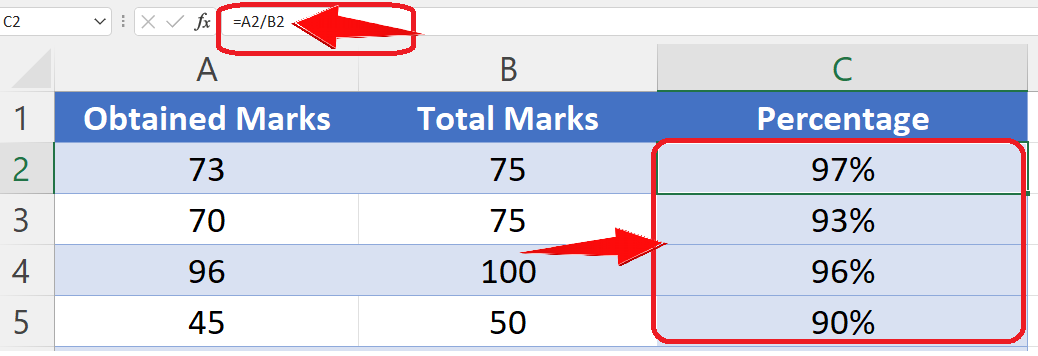# How to Paste Values Only in Excel(not formula) +shortcut

In such situations if you use the same command i.e. CTRL+C and then CTRL+V to copy data from those cells with formulas in them, then we will get incorrect results. In this tutorial we’ll learn the trick to copy the values only and avoid copying the formula by using special shortcut keys.

To learn this let’s look at an example where we have percentages calculated by percentage formula in Column C. Now follow along the simple steps to learn how to copy values only using shortcut keys.Anyone who has ever worked with windows must know about the famous shortcuts CTRL+C for copying values or files and CTRL+V for pasting the values or files. The same commands are used in Excel as well to copy data values and paste them in other cells. However, sometimes we come across situations where we use some specific formulas in order to get the required data.

### Step 1 – Select the cells with formulas– First select all the cells with formulas in them.
– Press CTRL+C to copy all cells as shown above.

### Step 2 – Paste the values using CTRL+ALT+V then U– After copying the cells with formulas, if you wish to paste the values to another range then select that range. Otherwise the new values will be pasted in the same cells.
– Now use the CTRL+ALT+V keyboard shortcut to open up paste special window.
– Press U to select values with number formats. The values will be pasted in the selected cells without the formula now as shown in the figure above.

So by using this paste special shortcut we can remove the formulas from cells and keep values only while keeping number formats.